# 6) Find the value of the inductor , if v.(t) = 10cos104 V and the average...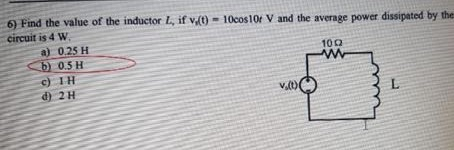6) Find the value of the inductor , if v.(t) = 10cos104 V and the average power dissipated by the circuit is 4 W a) 0.25 H b) 0.5 H c) 1H V.DO d) 2 H

This Homework Help Question: "6) Find the value of the inductor , if v.(t) = 10cos104 V and the average..." No answers yet.

We need 10 more requests to produce the answer to this homework help question. Share with your friends to get the answer faster!

0 /10 have requested the answer to this homework help question.

Once 10 people have made a request, the answer to this question will be available in 1-2 days.
All students who have requested the answer will be notified once they are available.

#### Earn Coin

Coins can be redeemed for fabulous gifts.

Similar Homework Help Questions
• ### A 10.7-V battery, a 4.98-Ω resistor, and a 10.4-H inductor are connected in series. After the...

A 10.7-V battery, a 4.98-Ω resistor, and a 10.4-H inductor are connected in series. After the current in the circuit has reached its maximum value, calculate the following.(a) the power being supplied by the battery W What is the maximum value of the current? What role does the inductor play when the current has a constant value?(b) the power being delivered to the resistor W You can determine the energy stored in the inductor from how much energy would be...

• ### 4. In the circuit below, V = 120 V, w = 200 rad/s, L = 0.500...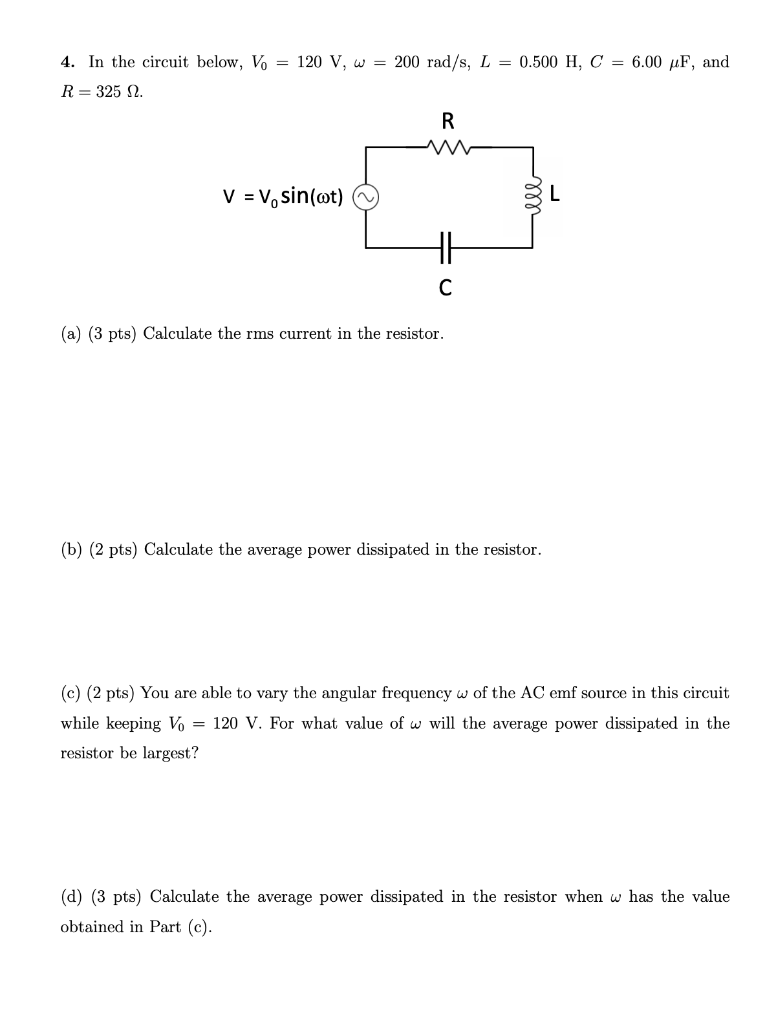4. In the circuit below, V = 120 V, w = 200 rad/s, L = 0.500 H, C = 6.00 uF, and R= 325 2. R w V = V, sin(ot) HE с (a) (3 pts) Calculate the rms current in the resistor. (b) (2 pts) Calculate the average power dissipated in the resistor. (c) (2 pts) You are able to vary the angular frequency w of the AC emf source in this circuit while keeping Vo = 120 V....

• ### Ch. 23: I The 5.05 A through a 1.50 H inductor is dissipated by a 2.10...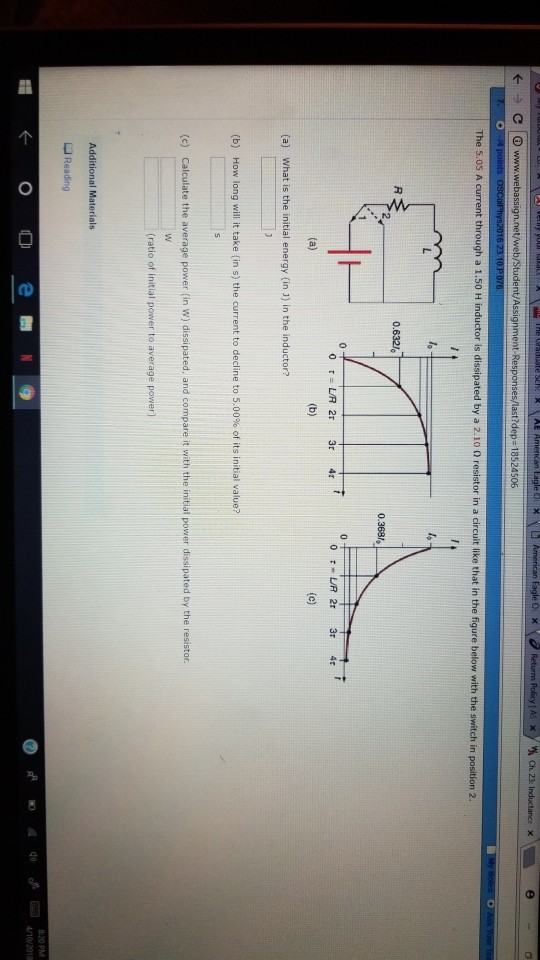Ch. 23: I The 5.05 A through a 1.50 H inductor is dissipated by a 2.10 0 resistor in a circuit like that in the figure below with the switch in position 2 0.632«? (a) What is the initial energy (in 1) in the inductor? (b) How long will it take (in s) the current to decline to 5.00% of its initial value? (c) Calculate the average power (in w) dissipated, and compare it with the initial power dissipated by...

• ### 0.500 H, C = 6.00 pF, and 4. In the circuit below, Vo = 120 V,...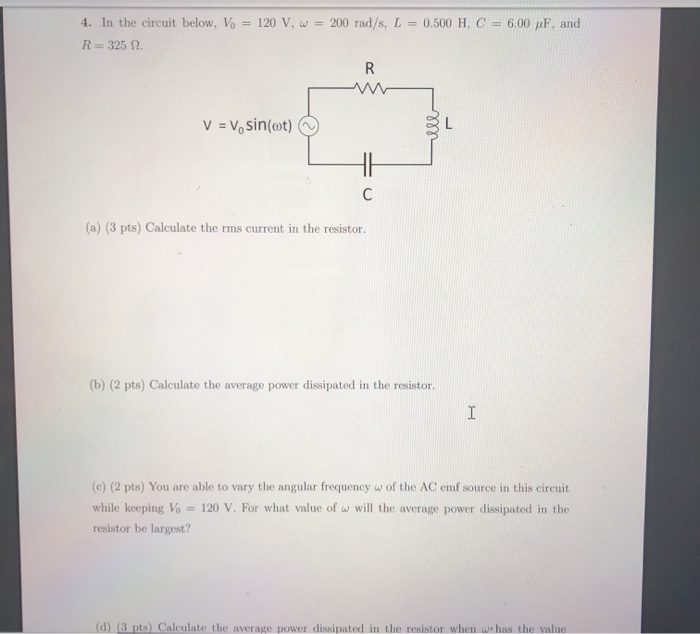0.500 H, C = 6.00 pF, and 4. In the circuit below, Vo = 120 V, W = 200 rad/s, L R= 325 2. R V = V, sin(ot) C (a) (3 pts) Calculate the rms current in the resistor. (b) (2 pts) Calculate the average power dissipated in the resistor. I (c) (2 pts) You are able to vary the angular frequency w of the AC emf source in this circuit while keeping Vo = 120 V. For what...

• ### What is the average power dissipated in the lightbulb when L = 0? The inductor is...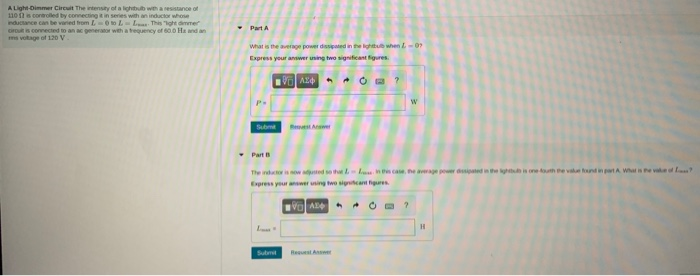What is the average power dissipated in the lightbulb when L = 0? The inductor is now adjusted so that L = L max. In this case the average power dissipated in the lightbulb is one fourth the value found in part A. What is the value of Lmax? A Ligh-Dimmer Circuit The intensity of a light with a resistance 110 is controlled by connecting in series with an inductor whose inductance can be vied from LLL. This lighter Drois...

• ### 2. In the circuit below all resistors are 1-ohm and the value of the inductor is 1H a. Determine the transfer function 'e in the frequency domain. b. If Vi(t) = 3 n= 1-sin (nrt) determine vo(t) c...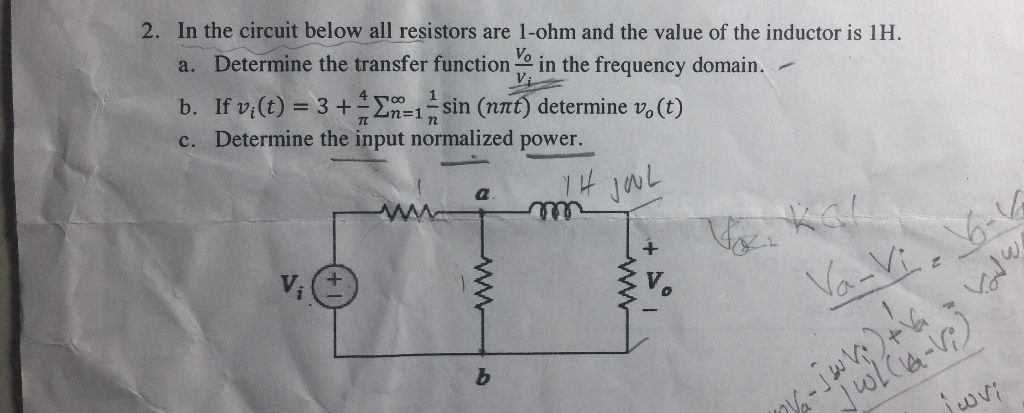2. In the circuit below all resistors are 1-ohm and the value of the inductor is 1H a. Determine the transfer function 'e in the frequency domain. b. If Vi(t) = 3 n= 1-sin (nrt) determine vo(t) c. Determine the input normalized power 1 나 1 V. 2. In the circuit below all resistors are 1-ohm and the value of the inductor is 1H a. Determine the transfer function 'e in the frequency domain. b. If Vi(t) = 3 n=...

• ### 4. In the circuit below, Vo = 120 V, w = 200 rad/s, L = 0.500...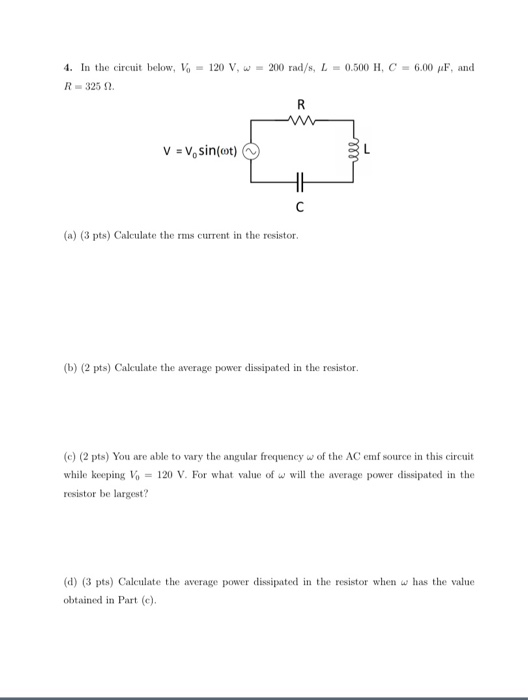4. In the circuit below, Vo = 120 V, w = 200 rad/s, L = 0.500 H, C = 6.00 pF, and R-3252 R V = V, sin(at) HE с (a) (3 pts) Calculate the rms current in the resistor. (b) (2 pts) Calculate the average power dissipated in the resistor. (e) (2 pts) You are able to vary the angular frequency w of the AC emf source in this circuit while keeping Vo = 120 V. For what value...

• ### A 22.0-mH inductor, with internal resistance of 22.0 Ω, is connected to a 110-V rms source....

A 22.0-mH inductor, with internal resistance of 22.0 Ω, is connected to a 110-V rms source. If the average power dissipated in the circuit is 62.0 W, what is the frequency? (Model the inductor as an ideal inductor in series with a resistor.)

• ### A sinusoidal voltage V(t) = (80.0V)sin(500t) is applied to a series RLC circuit with L= 700...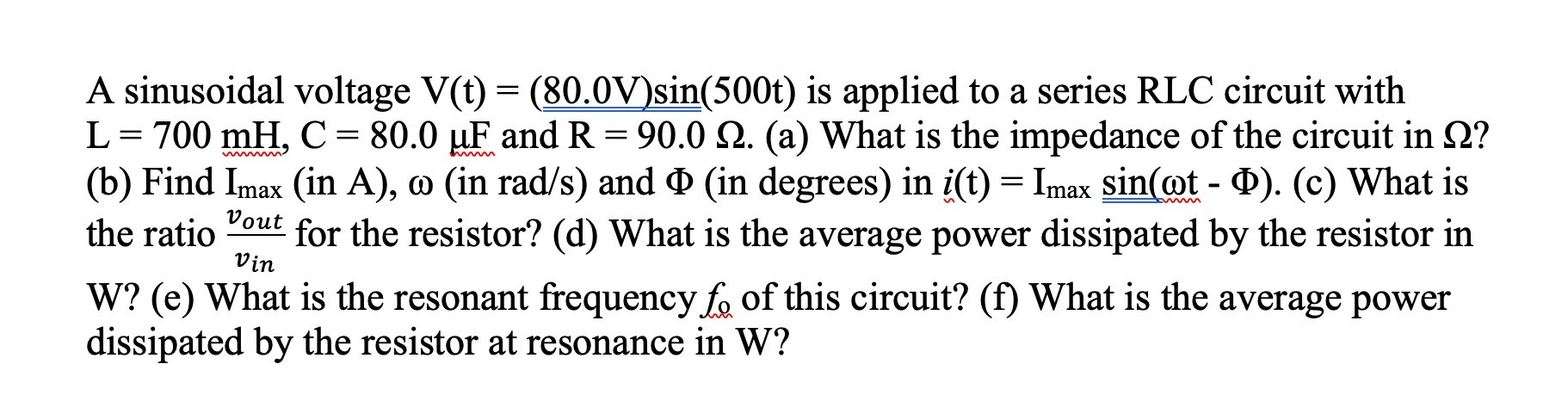A sinusoidal voltage V(t) = (80.0V)sin(500t) is applied to a series RLC circuit with L= 700 mH, C = 80.0 uF and R = 90.0 12. (a) What is the impedance of the circuit in 2? (b) Find Imax (in A), o (in rad/s) and Q (in degrees) in i(t) = Imax sin(@t-0). (c) What is the ratio Pout for the resistor? (d) What is the average power dissipated by the resistor in W? (e) What is the resonant frequency...

• ### Please do the problem if you can do ALL parts. t-0 a SW1 SW2 0.5 Ω 2 1Ω V. R3 20 A T v(t) 0.5 F 0.5 H 0 Find the initial current i(0) through the inductor and the initial voltage v(0) across the capa...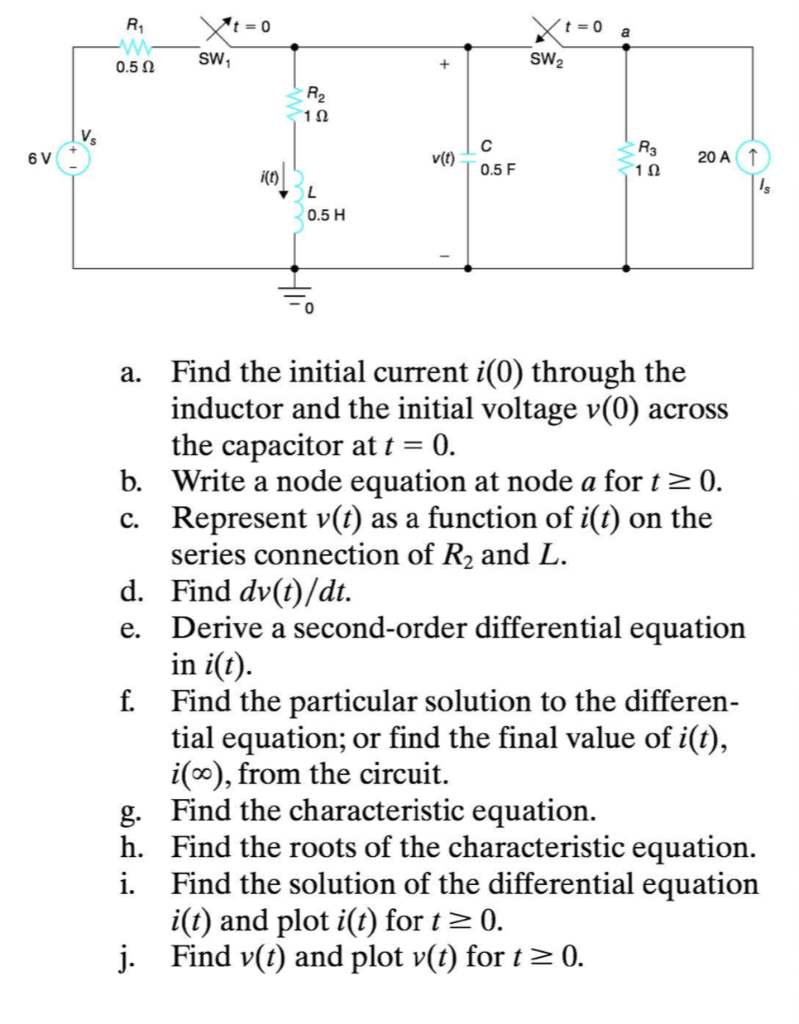Please do the problem if you can do ALL parts. t-0 a SW1 SW2 0.5 Ω 2 1Ω V. R3 20 A T v(t) 0.5 F 0.5 H 0 Find the initial current i(0) through the inductor and the initial voltage v(0) across the capacitor at t 0. b. Write a node equation at node a fort2 0. c. Represent v(t) as a function of i(t) on the series connection of R2 and L. Find dv(t)/dt. Derive a second-order differential...